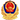# 2015年GMAT模拟试题及答案(数学)

2015-08-19 15:59:00 无忧考网2015年GMAT模拟试题及答案(数学)

考生若想在GMAT数学考试中取得好成绩，首先要掌握GMAT数学词汇和基本概念，这样才能又快又好完成GMAT数学题。

1.一枚硬币向上抛，两面的机率各为一半，问连抛三次，同一面的机率。(1/4)

同一面有两种情况：故1/8+1/8=1/4

2. 11球，6红，5蓝，without replacement, 问取一蓝一红的possibility. 6/11

第一次取蓝的情况 C15*C16 .第一次取红的情况: C16*C15， 总共的情况为C111C110=110 则概率为 60/110=6/11

3. 大于700的三位整数中，有多少是奇数，要求每个数字都不为零，且每位数字都不同。答案： 91 How to get the answer?

4. is xy>z? 我觉得是C

(1). xyz=1 (2). xyz^2>1 KEY: e why not C? (as NOT is also an answer)

5.X,Y,Z是三角形的三个边, 且X2^1/2)

.1/2XYsin(a)=1 而1/2XYsin(a)<1/2Y^2sin(a)

故1<1/2Y^2sin(a) => Y^2>2/sin(a)>2

所以 Y>2^1/2

6.

O

X X

X X X

X X X X

X X X X X

M M M M

上图第一行"O"表一小球, 第2,3,4行的"X"表障碍物, 第6行表小槽. 小球从上落下,在第三行时

受到障碍物的阻止, 其向左或右的机率相等, 各占50%. 以下依然. 问最后球掉到第六行的第二个

M的机率? (3/8)

对每一行落到X的概率都表示出来，然后即可得出结论为3/8

7. TWO KEYS， 放到已有5把钥匙chain中，问这两把钥匙相邻的概率 ?

NO.3 应该是环形的. 上面答案是机井上的. 我的答案是5/P2,10 = 1/9 不知对吗?在环形CHAIN上, 共有五个空位, 每个可放一个或二个KEY, 可得P2,10

相临情况为五个.得到1/9

8.给出标准方差公式，有一组数1，3，5，7。。。19 ，现在变动一下，问新表准方差A，B，C，D，E的MEAN是16，E为40，且E>D>C>B>A， 求C的可能值?

标准差的公式为:

sqrt((a1-a)^2+(a2-a)^2+...+(an-a)^2) 其中a=(a1+a2+..+an)/n，。怎么变动?你没说啊

9.某餐厅有2种不同水果, 6种不同蛋糕, 若餐后甜品每次都以a same number of kinds of fruit and cake, 则该餐厅有几种餐后甜点? 根据我的理解, C2,1C6,1+C2,2C6,2=12+15=27。27为选项E。选项B为12。

10. 某个公司职员大于10人小于40人, 开会时若每4人用一个桌子, 余3人独用一个; 若每5人用一个, 则余3人独用一个. 问现6人用一个桌子, 将余几个独用一个桌子.

这题比较简单。 这么考虑，人数被4除余3，被5除余3，问被6除余几?

10<4a+3<40 10<5b+3<40 则此数为: 23 则被6除余5

11. If x and y are positive integers such that x=8y+12,what is the greatest common divisor of x and y?

1) x=12u,where u is an integer

2) y=12z,where z is 解答：由题知: x=4(2y+3)

由(1)知: x=12u 则y=3k k is an integer. x=12(2k+1) 则x,y公因子不能确定.

由(2)知: y=12z 则x=12(6z+1) ,因此x,y的公因子是12。

这题我认为选Ban integer

12. 2^100-22^96, 问其的质因子是几?(答案为5)

2^100-22^96,肯定是题目有误，可能是2^100-2^96. 2^96(2^4-1)------2^96*3*5, 因此为5。

13. The possibility that the value of Stock A will increase is 0.34 and the possibility that stock B will increase is0.68 What is the biggest possibility that neither will happen? (I am not sure about the numbers.)

I am not very sure if I was correct for this question, therefore I prefer not to mislead you. However, the answer is absolutely not (1-0.34)*(1-0.68).

概率P=满足某个条件的所有可能情况数量/所有可能情况数量

性质 0<=P<=1

a1,a2为两两不相容的事件(即发生了a1，就不会发生a2)

P(a1或a2)=P(a1)+P(a2)

a1,a2不是两两不相容的事件，分别用集合A和集合B来表示

即集合A与集合B有交集，表示为A*B (a1发生且a2发生)

集合A与集合B的并集，表示为A U B (a1发生或a2发生)

则

P(A U B)= P(A)+P(B)-P(A*B)。。。。。。。。。。。。。。。。。公式2

还有就是条件概率：

考虑的是事件A已发生的条件下事件B发生的概率

定义：设A，B是两个事件，且P(A)>0,称

P(B|A)=P(A*B)/P(A)

GMAT试题相关推荐京公网安备 11010802026788号